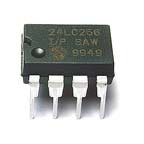# EEPROM and Arduino. A deeper viewIn this tutorial we will discuss a little deeper about the EEPROM, "our hard disk", than the "arduino and memory" generic article posted before.
We will try to overcome the per-byte storage limitation of the EEPROM bundled library.
In the future we will also discuss a little more about the arduino datatypes.
Lets focus at the EEPROM library  for now. As you can see the read function returns the value stored in a certain location (byte).

The same is valid for the write function
EEPROM.write(address, value); //Where address is the the location to wirite in int and value is the byte to write.

As we can see we can read and write to the EEPROM byte values.

From our article binary numeral systems we know that a byte stores an 8-bit unsigned number, from 0 to 255.
So how we could store the integer value of 500 for example at the EEPROM ?
Directly simply you can't !
An unsigned integer is infact a 2 byte value. So has a useful range of 0 to 65,535  since 216 - 1 = 65,535 (65,536 elements in total).
How can we fit in a single byte a two byte information (unsigned int) ?
Someone could say 500/2=250 so i can store 250 and the multiply this per two in order to refind the correct value.
This method is not elegant at all.
If we see the arduino and memory article we can see that the EEPROM is an array of bytes.
Another not elegant method is to say ok... 255 values per byte... but since i have more bytes i can do 255+245=500 (using two bytes) in order to store the 500. Yes but what if we wanted to store the 1000? We will need 4 bytes.
This method is not efficient at all, knowing that every unsigned int occupies only two bytes.

So in order to store an unsigned integer we just have to use two bytes from the EEPROM.

Save should take the unsigned integer broke it into two bytes and store these two bytes into two different byte cells of the EEPROM.
The read function will do the opposite. Goes into two consecutive cells of the EEPROM reads the two bytes and fuse them into a single "two byte" element (unsigned int).

To do this trick we need to know the bitwise operators discussed in the article "bitwise operators and tricks" .
If you do not know of what we are talking about go ahead and read that article first and then come back here.

The save function do something like this :
void SaveToEEPROM(unsigned int MyInteger)
We have as input an unsigned integer MyInteger. Lets say MyInteger=XXXXXXXXYYYYYYYY .
XXXXXXX is the left part and YYYYYYYY is the right part.
Notice that MyInteger is a two byte value (unsigned int) so has 16 bits.
The trick is to break MyInteger into a two pseudo-byte values (unsigned int) wl and wr (left and right part).
We can define two temp unsigned integers one that has only the left part of MyInteger wl=00000000XXXXXXXX (l means left part)
and wr is the right part of the MyInteger 00000000YYYYYYYY.
The wl=MyInteger>>8 so wl=00000000XXXXXXXX
The wr=MyInteger<<8 give as wr=YYYYYYYY00000000 and then the wr=wr>>8 gives wr=00000000YYYYYYYY
Now using
EEPROM.write(0,wl); //I write XXXXXXXX at the address 0
EEPROM.write(1,wr); //I write YYYYYYYY at the address 1
and we are done.
Notice that EEPROM.write writes only the last 8bits of the wl/wr but infact only there is located our information. In fact :
wl=00000000XXXXXXXX=XXXXXXXX
wr=00000000YYYYYYYY=YYYYYYYY